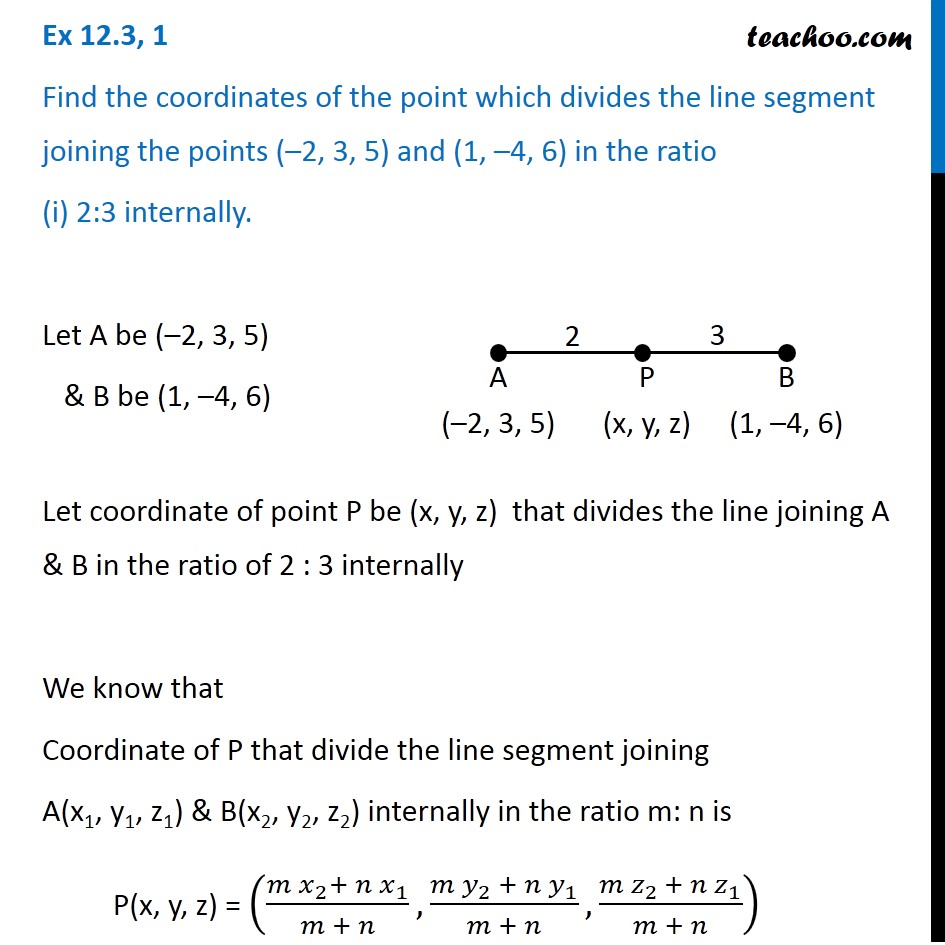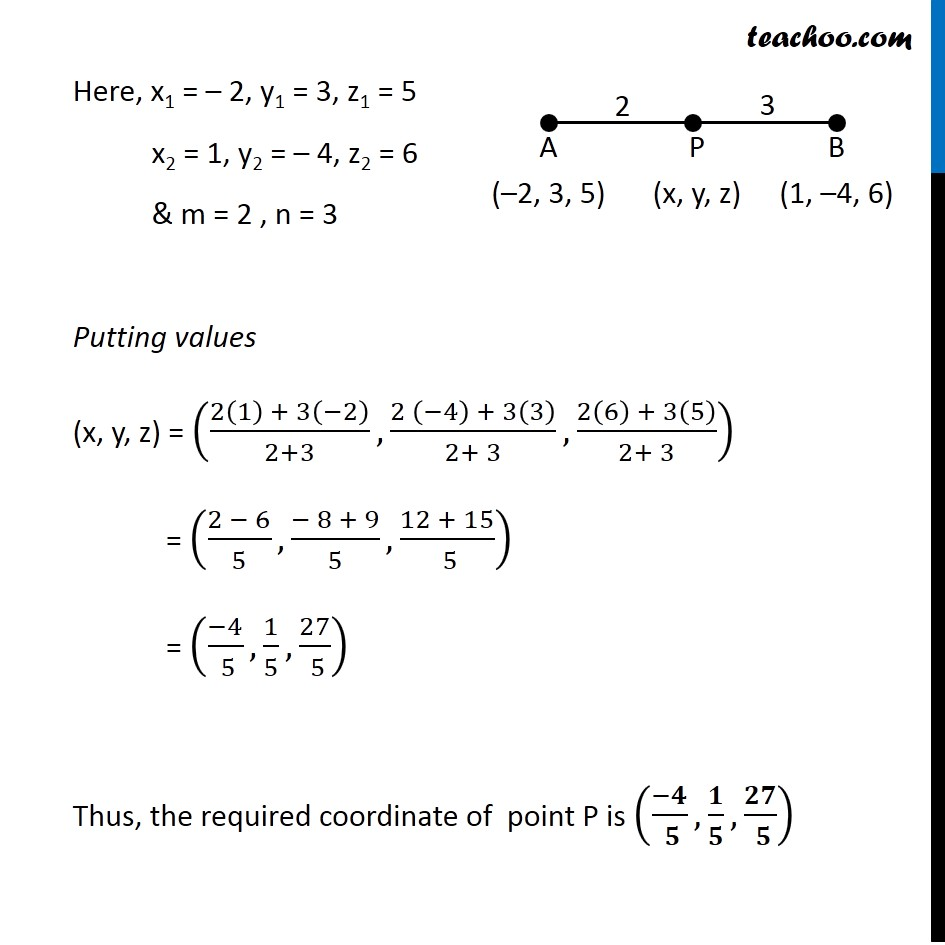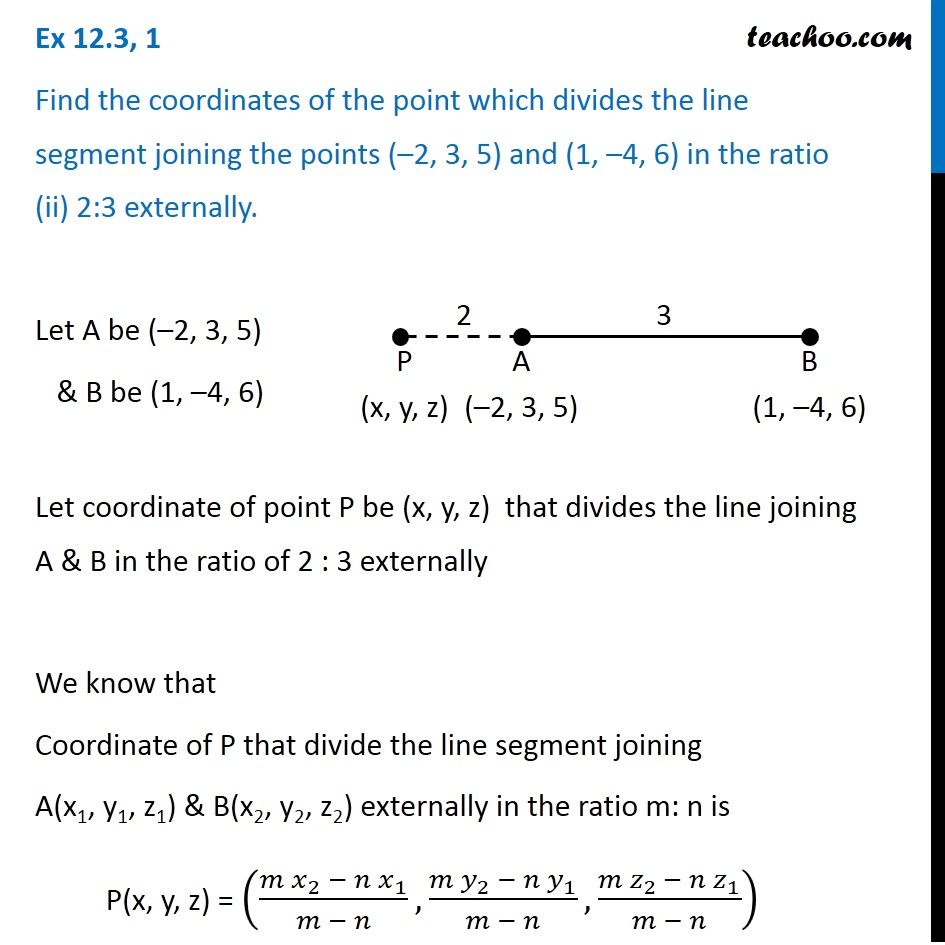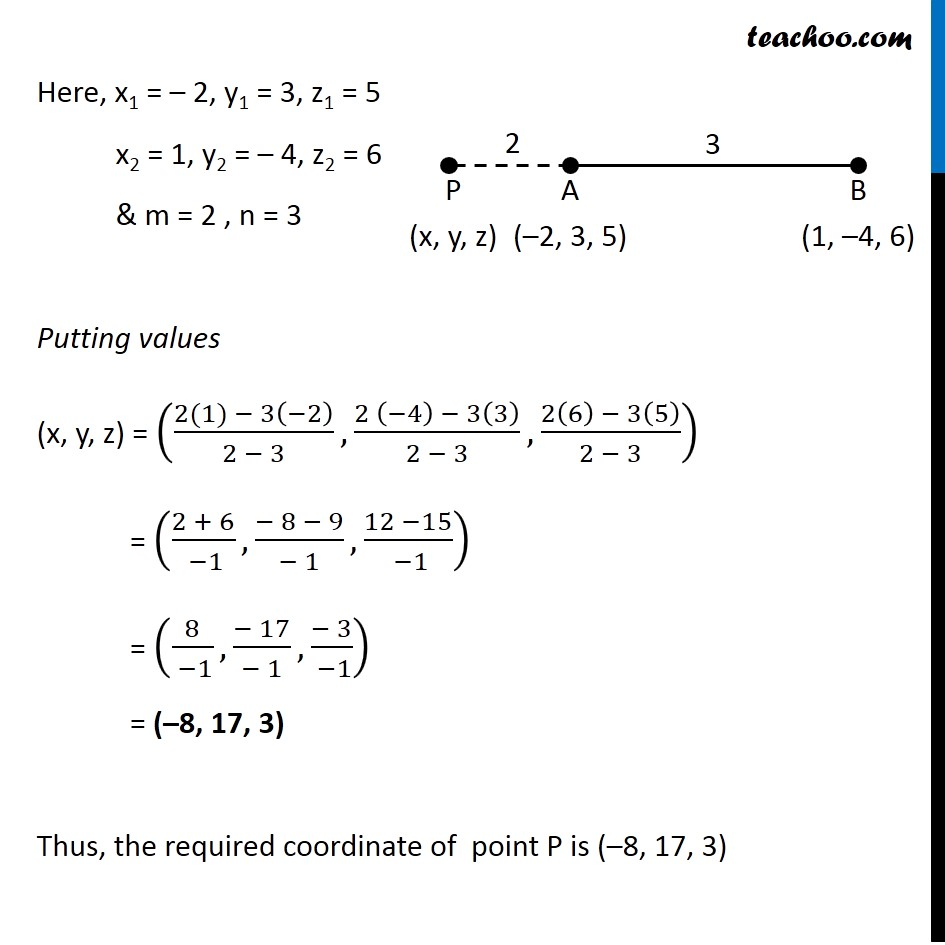Subscribe to our Youtube Channel - https://you.tube/teachoo

1. Chapter 12 Class 11 Introduction to Three Dimensional Geometry
2. Serial order wise
3. Ex 12.3

Transcript

Ex 12.3, 1 Find the coordinates of the point which divides the line segment joining the points (–2, 3, 5) and (1, –4, 6) in the ratio (i) 2:3 internally. Let A be (–2, 3, 5) & B be (1, –4, 6) Let coordinate of point P be (x, y, z) that divides the line joining A & B in the ratio of 2 : 3 internally We know that Coordinate of P that divide the line segment joining A(x1, y1, z1) & B(x2, y2, z2) internally in the ratio m: n is P(x, y, z) = ((〖𝑚 𝑥〗_2+〖 𝑛 𝑥〗_1)/(𝑚 + 𝑛),(〖𝑚 𝑦〗_2 +〖 𝑛 𝑦〗_1)/(𝑚 + 𝑛),(〖𝑚 𝑧〗_(2 )+〖 𝑛 𝑧〗_1)/(𝑚 + 𝑛)) Here, x1 = – 2, y1 = 3, z1 = 5 x2 = 1, y2 = – 4, z2 = 6 & m = 2 , n = 3 Putting values (x, y, z) = ((2(1) + 3(−2))/(2+3),(2 (−4) + 3(3))/(2+ 3),(2(6) + 3(5))/(2+ 3)) = ((2 − 6)/5,(− 8 + 9)/5,(12 + 15)/( 5)) = ((−4)/( 5),1/5,27/( 5)) Thus, the required coordinate of point P is ((−𝟒)/( 𝟓),𝟏/𝟓,𝟐𝟕/( 𝟓)) Ex 12.3, 1 Find the coordinates of the point which divides the line segment joining the points (–2, 3, 5) and (1, –4, 6) in the ratio (ii) 2:3 externally. Let A be (–2, 3, 5) & B be (1, –4, 6) Let coordinate of point P be (x, y, z) that divides the line joining A & B in the ratio of 2 : 3 externally We know that Coordinate of P that divide the line segment joining A(x1, y1, z1) & B(x2, y2, z2) externally in the ratio m: n is P(x, y, z) = ((〖𝑚 𝑥〗_2 −〖 𝑛 𝑥〗_1)/(𝑚 − 𝑛),(〖𝑚 𝑦〗_2 −〖 𝑛 𝑦〗_1)/(𝑚 − 𝑛),(〖𝑚 𝑧〗_(2 )−〖 𝑛 𝑧〗_1)/(𝑚 − 𝑛)) Here, x1 = – 2, y1 = 3, z1 = 5 x2 = 1, y2 = – 4, z2 = 6 & m = 2 , n = 3 Putting values (x, y, z) = ((2(1) − 3(−2))/(2 − 3),(2 (−4) − 3(3))/(2 − 3),(2(6) − 3(5))/(2 − 3)) = ((2 + 6)/( −1),(− 8 − 9)/(− 1),(12 −15)/( −1)) = (8/( −1),(− 17)/(− 1),(− 3)/( −1)) = (–8, 17, 3) Thus, the required coordinate of point P is (–8, 17, 3)

Ex 12.3